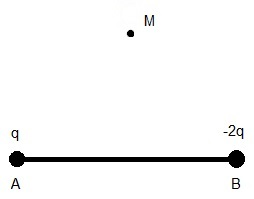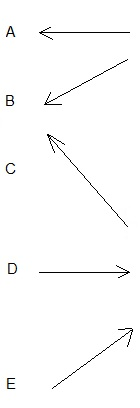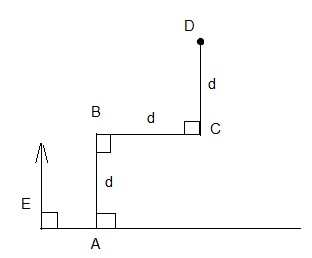# Free SAT II Physics Practice Questions with Solutions Electric Fields and Forces

 Sat Physics subject questions on electric fields and forces with detailed solutions, similar to the questions in the SAT test are presented. Answers at the bottom of the page. If vector F is the force between two charges q1 and q2, then what is the force between the charges 2q1 and -3q2 located at the same position as q1 and q2 respectively? A) F B) 6 F C) - F D) - 6 F E) 2 F 36 J is used to move a 3 coulombs through a potential difference of V. What is V? A) 1/12 v B) 12 v C) 78 v D) 39 v E) 33 vFig1. - Electric Field On Perpendicular Bisector. What is the direction of the electric field at point M due to the charges q and -2q at point A and B such that M is a point on the perpendicular bisector of AB and charge q is negative?Fig3. - Work by Electric Field. What is the work done by the electric field E (assumed constant and present everywhere) to move charge q, starting from point A, along path ABCD as shown in figure 3? A) q E d B) 3 q E d C) 0 D) 2 q E d E) 4 q E d The potential difference is defined as a measure of A) work done per unit charge B) Force per unit charge C) power per unit charge D) electric field per unit charge E) electric current Which of the following is correct about a closed electric circuit including a battery and a resistor? A) charges move through the circuit because the resistor supplies energy. B) charges move through the circuit because the battery maintains an electric potential difference across the ends of the external circuit. C) charges move because they are supplied by the battery. D) charges move through the circuit because they can acquire kinetic energy from the resistor. E) None of the above is correct. What is the work done on 5 electrons moving through a uniform electric field from a potential of 0 volts to a potential of 10 millivolts. The movement is parallel to the electric field. ( charge of electron = - 1.6 � 10-19 C) A) - 8 � 10-18 J B) -1.6 10-19 J C) - 8 � 10-21 J D) 3.125 � 1017 J E) 3.125 � 1019 J The unit volt is defined as A) 1 joule / coulomb B) 1 coulomb / second C) 1 meter / coulomb D) 1 newton / coulomb E) 1 coulomb / joule Two large metallic plates of the same size are connected each to the terminals of a battery. The distance between the plates is uniform and equal to 1 cm. What is the intensity of the electric field between the plates if the potential difference between the terminals of the battery is equal to 10 volts?(The electric field between the plates is assumed uniform) A) 10 v/m B) 0.1 v/m C) 11 v/m D) 9 v/m E) 1000 v/m The electric field at a distance r from a charge Q is equal to E. What is the electric field at a distance 2 r from a charge 2 Q? A) E B) 2 E C) E / 2 D) 4 E E) E / 4 Answers to the Above questions D B C D A B C A E C